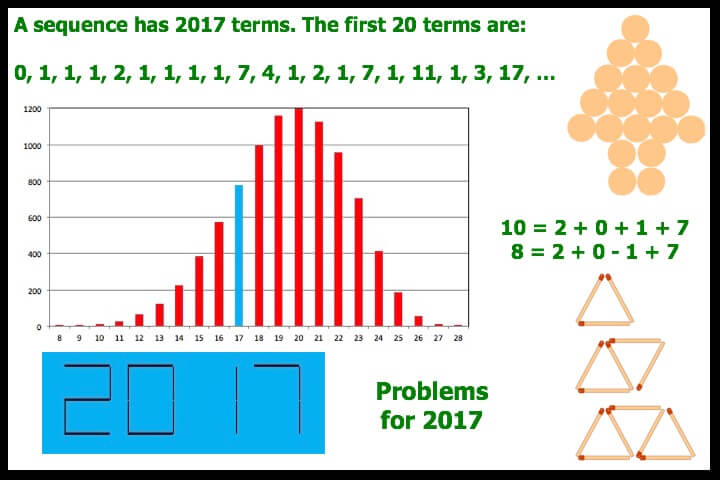Ideas for problem solving and mathematical reasoning using 2017 including:

• what is the smallest number that cannot be made with exactly one each of 2, 0, 1 and 7: depends on what is allowed – here 1 to 37 can be made
• in calculator display as above, 16 of 28 possible ‘lines’ are used to make the four digits: how many other 4 digit numbers from 1000 to 9999 also use 16 ‘lines’?
• which, ‘match’ sequences (one is shown above) have a term with 2017 matches in it?
• if you make a fir tree, as above with exactly 2017 coins what combinations of triangular ‘tree tops’ and ‘rectangular trunks’ make a ‘valid’ tree?
• identify the 2017 term sequence which has the first 20 terms given above.